﻿ 云南省能源效率空间差异及影响因素分析

# 云南省能源效率空间差异及影响因素分析Spatial Difference of Energy Efficiency and Its Influencing Factors in Yunnan Province

Abstract: This paper analyzes the energy efficiency of Yunnan Province in 2005-2014, reveals its spatial and temporal differences and its influencing factors, and further provides suggestions for improving the energy efficiency level of Yunnan Province and the overall coordinated development of the energy industry in Yunnan Province. The results show that: 1) From the time point of view, the overall energy efficiency of Yunnan Province is gradually increasing with time, but the difference in energy efficiency between the states and the states is expanding; 2) From the perspective of space exploration results, the space club phenomenon of energy efficiency in Yunnan Province is not significant, that is, there is no significant spatial spillover effect on energy efficiency between Yunnan and Guizhou; 3) Power consumption level, technological progress and economic development level in Yunnan Province play crucial roles in the improvement of energy efficiency. The deepening of the government’s influence is not conducive to the improvement of energy efficiency in Yunnan Province. The impact of opening up and industrial structure on energy efficiency is not significant.

1. 引言

2. 模型方法及指标数据

2.1. DEA模型

DEA方法是用数学规划模型来评价相同类型的评价单元(DMU)是否技术有效的一种非参数统计方法  ，其理论基础来源于Farrell提出的包络思想  。DEA方法的第一个模型(即CCR-DEA模型)在1978年由Cooper、Charnes和Rhodes三位著名运筹学家首先提出  ，经过四十年的发展，DEA理论日趋完善，方法也逐步成熟。DEA方法最早于80年代末被魏权龄引入中国  ，之后国内许多学者都对DEA方法进行了拓展研究。目前已经成为管理学、评价技术中重要的分析工具，并在许多研究领域中进行了成功的应用。

DEA方法的第一个模型也是最基本模型是CCR-DEA模型，这是一种规模报酬不变的DEA模型。假设有n个独立的决策单元：

${\mathrm{min}}_{\theta ,\gamma }\theta$

$s.t-{y}_{i}+{Y}_{\gamma }\ge 0$

${\theta }_{xi}-{X}_{\gamma }\ge 0$

2.2. 指标选取及数据来源

${K}_{i,t}={I}_{i,t}+\left(1-{\delta }_{i,t}\right){K}_{i,t-1}$

2.3. 结果分析Table 1. Total factor energy efficiency value of Yunnan province

3. 能源效率时空分异

3.1. 云南省各地州能源效率时间分异特征

${f}_{h}\left(x\right)=\frac{1}{n}\underset{i=1}{\overset{n}{\sum }}{K}_{h}\left(x-{x}_{i}\right)=\frac{1}{nh}\underset{i=1}{\overset{n}{\sum }}K\left(\frac{x-{x}_{i}}{h}\right)$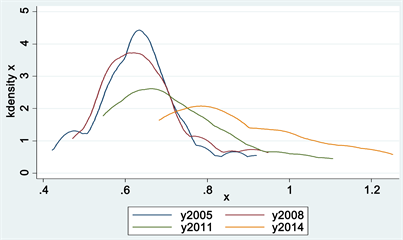Figure 1. Nuclear density distribution map of energy efficiency in Yunnan province for 4 years

3.2. 云南省各地州能源效率空间格局探索及演变规律

3.2.1. 云南省各地州能源效率空间格局探索

$Mora{n}^{\prime }s\text{}I=\frac{\underset{i=1}{\overset{n}{\sum }}\underset{j=1}{\overset{n}{\sum }}{W}_{ij}\left({X}_{i}-\stackrel{¯}{X}\right)\left({X}_{j}-\stackrel{¯}{X}\right)}{{S}^{2}\underset{i=1}{\overset{n}{\sum }}\underset{j=1}{\overset{n}{\sum }}{W}_{ij}}$Table 2. Global Moran’s I index of energy efficiency

3.2.2. 云南省各地州能源效率空间格局演变

1) 云南省能源效率总体并没有呈现显著的HH型和LL型的空间俱乐部现象，处于低低集聚的地区最多，高低集聚的地区几乎没有，而高高集聚的地区没有。

2) 低低集聚的地区主要分布在东部和南部地区，其他地区能源效率集聚类型不太稳定，其中能源效率集聚格局的变化主要为低高集聚区和高低集聚区的变化。在2005、2008、2011、2014年四个时间点上，集聚类型转移显著的为昆明，经历高低集聚类型向低低集聚类型的演变，能源效率的集聚类型在某一时刻发生重大转变的有大理和文山。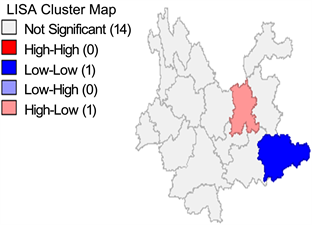(a) 2005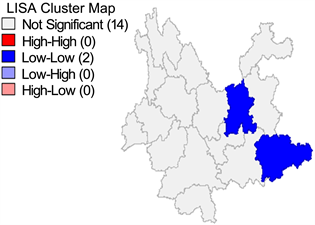(b) 2008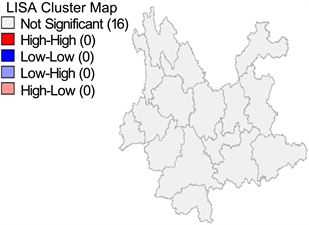(c) 2011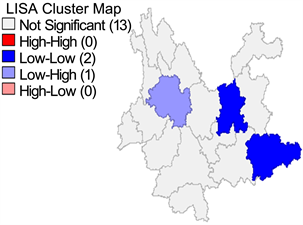(d) 2014

Figure 2. Evolution of local spatial relation patterns of energy efficiency

3.3. 云南省各地州能源效率差异性趋势

$CV=\frac{S}{\stackrel{¯}{x}}=\frac{\sqrt{\frac{{\sum }_{i=1}^{n}{\left({x}_{i}-\stackrel{¯}{x}\right)}^{2}}{n}}}{\stackrel{¯}{x}}$

$Gini=\frac{1}{2{n}^{2}\stackrel{¯}{x}}\underset{i=1}{\overset{n}{\sum }}\underset{j=1}{\overset{n}{\sum }}|{x}_{i}-{x}_{j}|$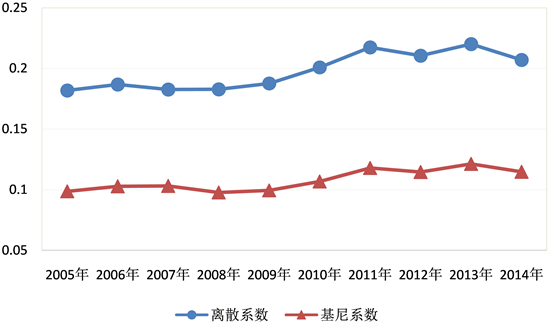Figure 3. Gini coefficient and coefficient of variation of energy efficiency in Yunnan provinces from 2005 to 2014

2005~2014年间，云南省各地州能源效率基尼系数在0.09~0.13之间徘徊，整体呈现较小幅度的波动上升态势(如图3)。具体而言就是基尼系数由2005年0.098波动上升至2014年的0.115，10年间各地州能源效率年均值差异扩大1.6%，这说明云南省各地州能源效率差距有扩大趋势。

4. 云南省能源效率影响因素分析Table 3. Dynamic panel regression results of influencing factors of energy efficiency in Yunnan provinces

1) 产业结构(IS)

2) 经济发展水平(ED)

3) 能源消费结构(ES)

4) 政府影响力(GE)

5) 市场化水平(ML)

6) 技术水平(TE)

7) 对外开放水平(OP)

5. 结论与政策建议

1) 减少政府干预，加快推进国有企业改革

2) 发展清洁能源，优化能源消费结构

3) 加大人才引进力度，健全协调发展机制

 程钰, 任建兰, 陈延斌, 等. 中国环境规制效率空间格局动态演变及其驱动机制[J]. 地理研究, 2016, 35(1): 123-136.

 李治, 李国平. 城市能源效率分布特征影响因素研究-基于空间计量模型[J]. 城市发展研究, 2010, 17(6): 22-26.

 范凤岩, 雷涯邻. 北京市能源效率评价及其影响因素分析[J]. 科技管理研究, 2014, 34(322): 28-32.

 张庆芝, 何枫, 赵晓. 基于超效率DEA的我国钢铁产业能源效率研究[J]. 软科学, 2012, 26(2): 65-68.

 沈能. 空间溢出、门槛特征与能源效率的经济增长效应[J]. 中国人口.资源与环境, 2012, 22(5): 153-157.

 刘佳骏, 董锁成, 李宇. 产业结构对区域能源效率贡献的空间分析——以中国大陆31省(市、自治区)为例[J]. 自然资源学报, 2011, 26(12): 1999-2011.

 Farrell, M.J. (1957) The Measurement of Productive Efficiency. Journal of the Royal Statistical Series A, 120, 253-290.
https://doi.org/10.2307/2343100

 魏权龄. 评价相对有效性的方法[M]. 北京: 人民出版社, 1998.

 Charnes, A., Cooper, W.W. and Phodes, E. (1978) Measuring the Efficiency of DMU. European Journal of Operation Research, 2, 429-444.
https://doi.org/10.1016/0377-2217(78)90138-8

 Banker, R.D. (1984) Estimating Most Productive Scale Size Using Data Envelopment Analysis. European Journal of Operational Research, 17, 35-44.
https://doi.org/10.1016/0377-2217(84)90006-7

 师博, 沈坤荣. 政府干预、经济集聚与能源效率[J]. 管理世界, 2013(10): 6-18.

Top